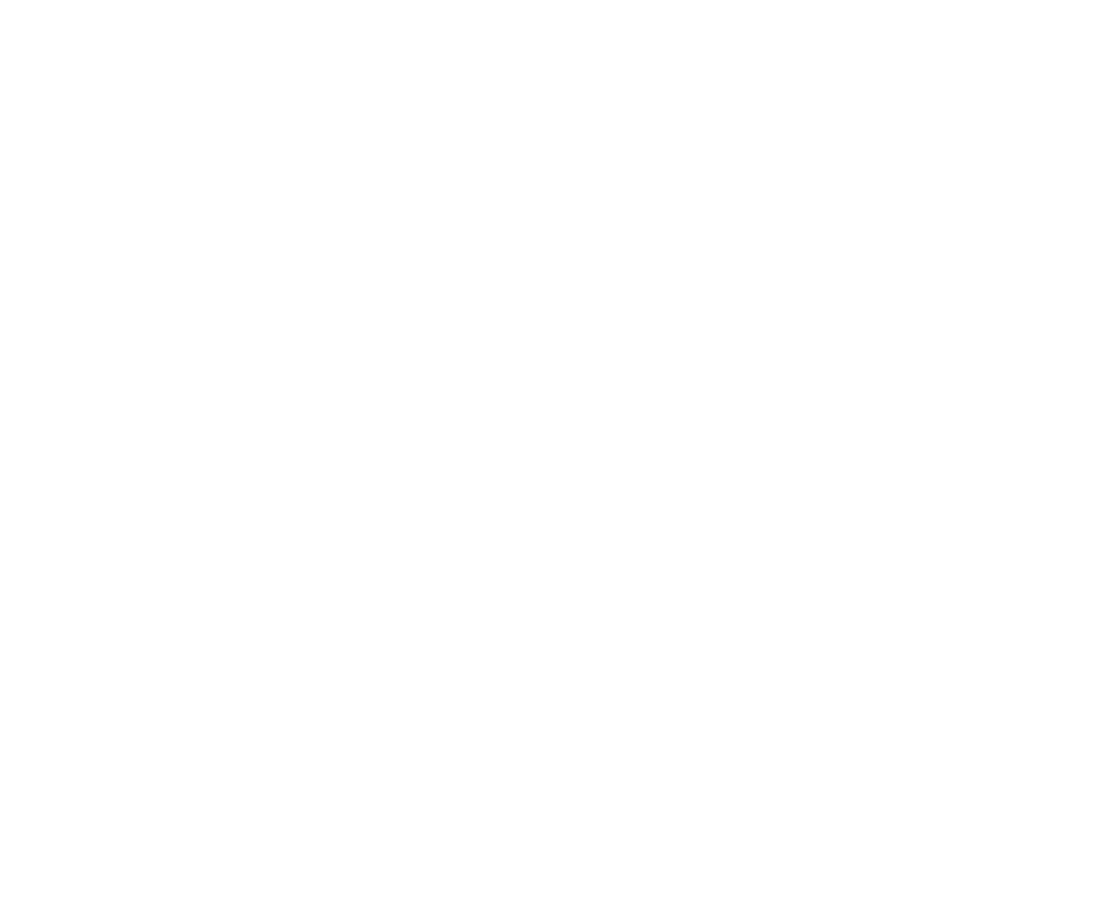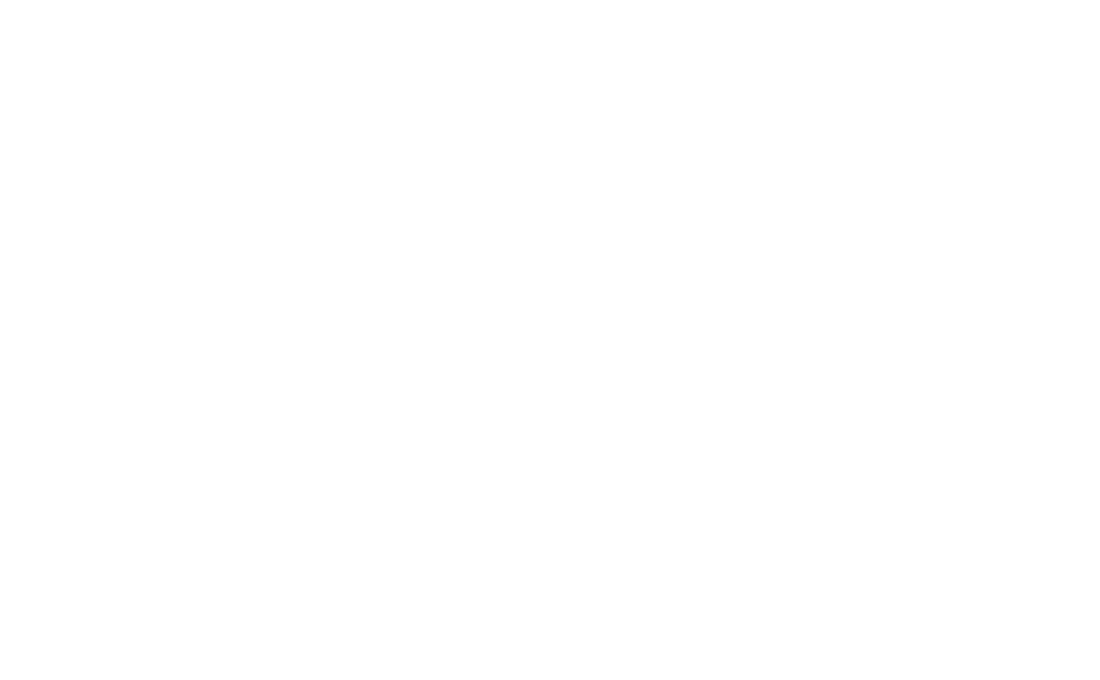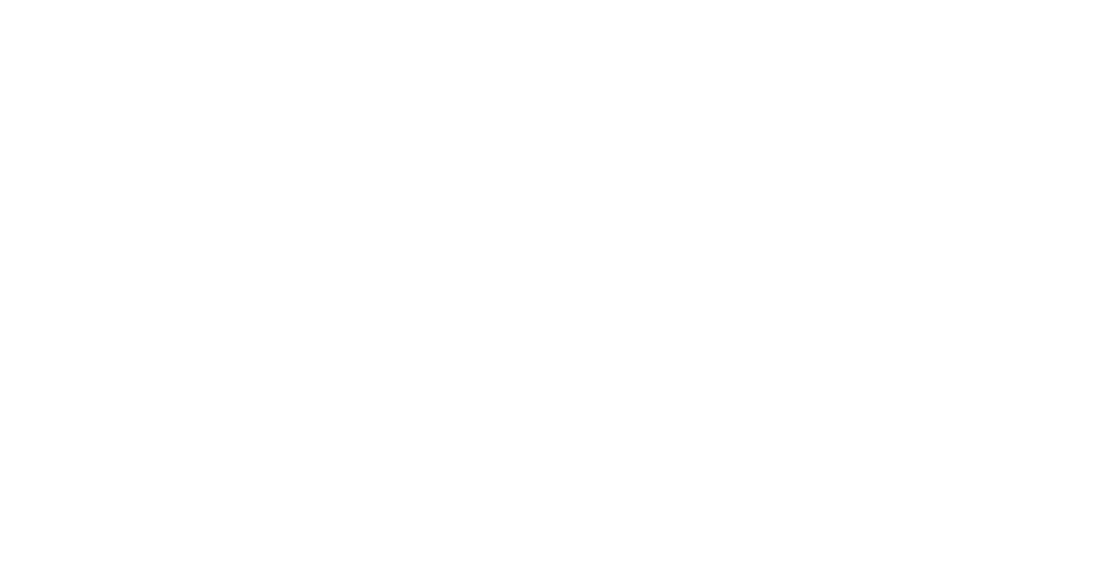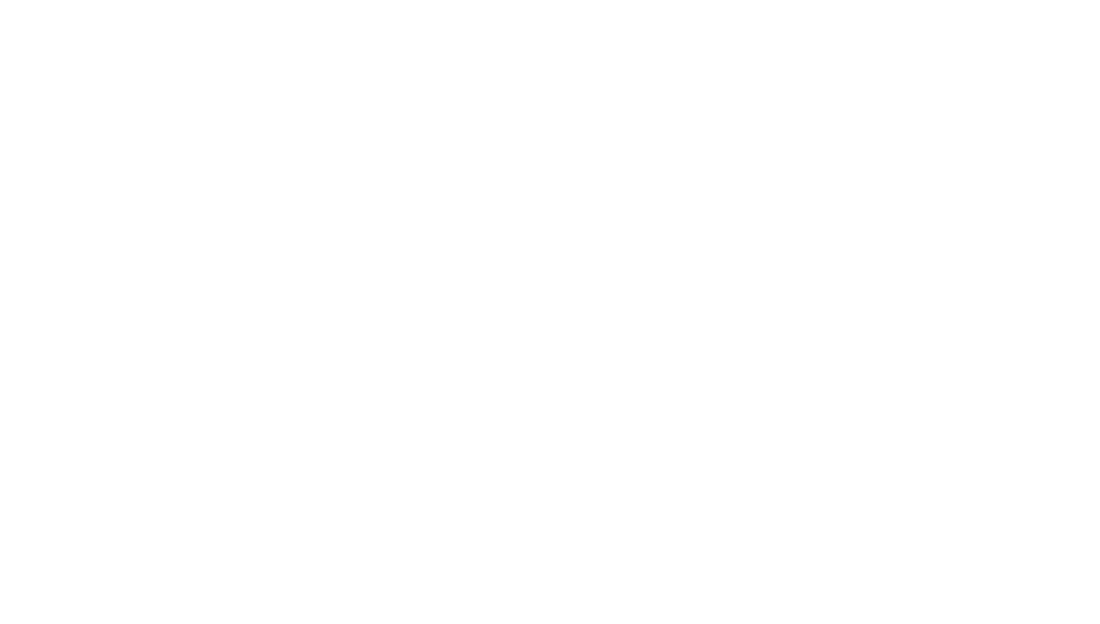# 4.15.1 3D Shapes - Surface Area

#### What is surface area?

• A face is one of the flat or curved surfaces that make up a 3D shape
• The surface area of a 3D shape is the sum of the areas of all the faces that make up the shape
• Note how we are carrying a 2D idea (area) into 3 dimensions here

#### Surface area – cuboids, pyramids, and prisms

• In cuboids, polygonal-based pyramids, and polygonal-based prisms (ie. pyramids and prisms whose bases have straight sides), all the faces are flat
• The surface area is found simply by adding up the areas of these flat faces
• When calculating surface area, it can be very helpful to draw a 2D net for the 3D shape in question
• For example:
• The base of a square-based pyramid is 15 cm on a side
• The triangular faces are identical isosceles triangles, each with a height (from the base to the top of the pyramid) of 23 cm
• Find the total surface area of the pyramid
• Draw a net for the shape:Area of square base =152 = 225 cm2
Area of one triangular face = ½ base × height = ½ × 15 × 23 =172.5 cm2
Total surface area =225 + 4 × 172.5 = 915 cm2

#### Surface area – cylinders, cones, and spheres

• All three of these shapes have curved faces, so we have to be a little more careful when calculating their surface areas

1. The net of a cylinder consists of two circles and a rectangle:• The total surface area of a cylinder with base radius r and height h is therefore given by:
Total surface area of a cylinder= 2πr2 + 2πrh

2. The net of a cone consists of the circular base along with the curved surface area:• The length l in that diagram is known as the slant height (while h is the vertical height of the cone)
• To find the surface area of a cone with base radius r and slant height l, we use the formulas:
Curved surface area of a cone = πrl
Total surface area of a cone = πr2+πrl

#### Exam Tip

The formula for the surface area of a sphere or the curved surface area of a cone will be given to you in an exam question if you need it. The rest of the formulas here come from what you should already know about areas of rectangles, triangles, and circles.

Be careful when calculating the surface area of a hemisphere:The total surface area consists of the curved part (half of a sphere) PLUS the flat circular face – so the total surface area is 3πr2

### Worked Example

CloseClose